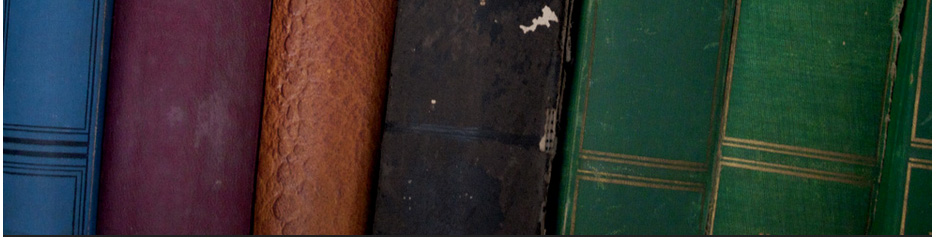## Secondary education# Algebra 1

 ks40001 Solving Simultaneous Equations by Elimination Example 1 ks40002 Simultaneous Equations – Elimination method Example 5 : ExamSolutions ks40003 Simultaneous Equations – Elimination method Example 4 : ExamSolutions ks40004 Simultaneous Equations – Elimination method Example 6 : ExamSolutions ks40005 Simultaneous Equations – Elimination method Example 2 : ExamSolutions ks40006 Completing the Square – Tutorial 2 : ExamSolutions ks41007 Completing the Square ks40008 Solving Quadratic Equations by the Square Root Method ks40009 Multiplying Square Roots – YourTeacher.com – Algebra Help ks4010 Simplifying Radicals – YourTeacher.com – Math Help ks4011 Quadratic Equations – Factoring and Quadratic Formula ks4012 Algebra Help – The Quadratic Formula ks4013 Solve Quadratic Equations using Quadratic Formula ks4014 Solving Linear Equations ks4015 Completing the square ks4016 Solving systems of equations – Substitution method ks4017 Math Help Quadratics: The Quadratic Formula ks4018 Algebra II – 3.3 Factoring Polynomials ks4019 intercepts and graphing linear equations ks4020 3 of 3 – How to graph y = mx + b (it’s easy) *with practice* ks4021 How to Find the Slope and y-Intercept by Writing Equation in Slope-Intercept ks4022 Intercepts and graphing linear equations ks4023 X and Y intercepts of a linear equation ks4024 Graphing Quadratic Equations ks4025 Finding the Minimum or Maximum of Quadratic Functions ks4026 How to Get the Equation of a Parabola given its intercepts and a point ks4027 How to Factor any Quadratic Equation ks4028 Finding the vertex and x-intercepts of a Quadratic Function ks4029 Basic Parabola ks4030 Logarithms 11 – Common and Natural Log
Ermias Home

HAREP The Horn of Africa Research and Knowledge Exchange Platform © 2010-2012

 Contact: rkidane@talk21.com Please click Here to send your feedback